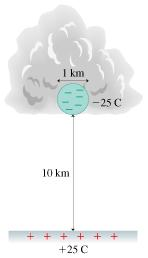# Problem: Storm clouds build up large negative charges, as described in the chapter. The charges dwell in charge centers, regions of concentrated charge. Suppose a cloud has -25 C in a 1.0-km-diameter spherical charge center located 10 km above the ground, as sketched in the figure. The negative charge center attracts a similar amount of positive charge that is spread out on the ground below the cloud.The charge center and the ground function as a charged capacitor, with a potential difference of approximately 4 x 108 m The large electric field between these two "electrodes" may ionize the air, leading to a conducting path between the cloud and the ground. Charges will flow along this conducting path, causing a discharge of the capacitor - a lightning strike.1) What is the approximate magnitude of the electric field between the charge center and the ground?A) 4 x 104 V/m                B) 4 x 105 V/mC) 4 x 106 V/m                D) 4 x 107 V/m2) What is the approximate capacitance of the charge center-ground system?A) 6 x 10-8 FB) 2 x 107 FC) 4 x 106 FD) 8 x 106 F3) If the cloud transfers all of its charge to the ground via several rapid lightning flashes lasting a total of 1.90 s, what is the average power?A) 12.7 GWB) 3.74 GWC) 2.74 GWD) 1.74 GW

###### FREE Expert Solution

Electric field:

$\overline{){\mathbf{E}}{\mathbf{=}}\frac{\mathbf{V}}{\mathbf{d}}}$

Charge stored in a capacitor:

$\overline{){\mathbf{Q}}{\mathbf{=}}{\mathbf{C}}{\mathbf{V}}}$

Energy stored in a capacitor:

$\overline{){\mathbf{U}}{\mathbf{=}}\frac{\mathbf{1}}{\mathbf{2}}{\mathbf{C}}{{\mathbf{V}}}^{{\mathbf{2}}}}$

Power:

$\overline{){\mathbf{P}}{\mathbf{=}}\frac{\mathbf{E}\mathbf{n}\mathbf{e}\mathbf{r}\mathbf{g}\mathbf{y}}{\mathbf{t}\mathbf{i}\mathbf{m}\mathbf{e}}}$

(1)

V = 4 × 108 V

d = 10 km = 10000 m

E = V/d = 4 × 108/10000 = 4 × 104 V/m

86% (86 ratings)###### Problem DetailsStorm clouds build up large negative charges, as described in the chapter. The charges dwell in charge centers, regions of concentrated charge. Suppose a cloud has -25 C in a 1.0-km-diameter spherical charge center located 10 km above the ground, as sketched in the figure. The negative charge center attracts a similar amount of positive charge that is spread out on the ground below the cloud.

The charge center and the ground function as a charged capacitor, with a potential difference of approximately 4 x 108 m The large electric field between these two "electrodes" may ionize the air, leading to a conducting path between the cloud and the ground. Charges will flow along this conducting path, causing a discharge of the capacitor - a lightning strike.

1) What is the approximate magnitude of the electric field between the charge center and the ground?

A) 4 x 104 V/m                B) 4 x 105 V/m

C) 4 x 106 V/m                D) 4 x 107 V/m

2) What is the approximate capacitance of the charge center-ground system?

A) 6 x 10-8 F

B) 2 x 107 F

C) 4 x 106 F

D) 8 x 106 F

3) If the cloud transfers all of its charge to the ground via several rapid lightning flashes lasting a total of 1.90 s, what is the average power?

A) 12.7 GW

B) 3.74 GW

C) 2.74 GW

D) 1.74 GW